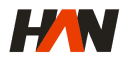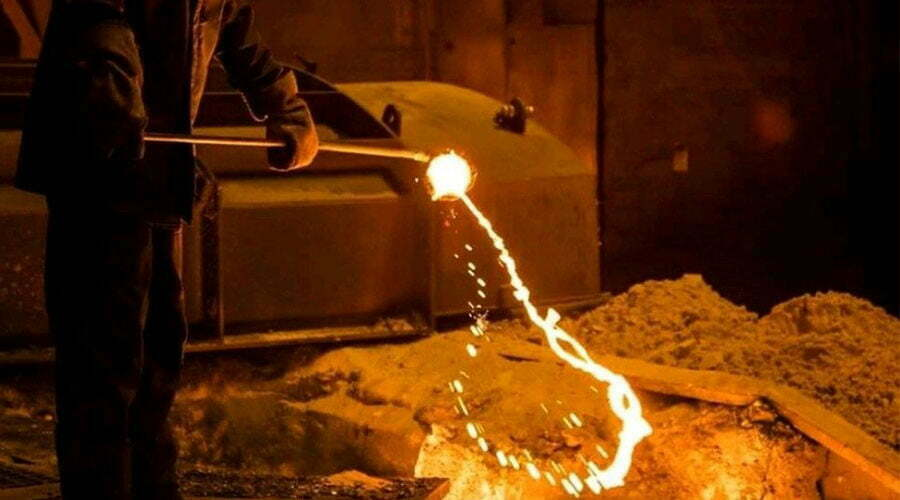# The Consumption of Foundry Melting FurnaceIn the operation of the foundry melting furnace, what are the main aspects of the loss of output power? From the design and calculation of the induction furnace, it can be seen that only a part of the active power sent from the power supply is absorbed by the charge, and the rest of the energy becomes various forms of loss. In order to improve the technical and economic indicators of the furnace, the designers and operators of the furnace should understand the energy consumption of each part and its influencing factors, so as to take measures to minimize various losses.

The only useful power in an induction furnace is the part that heats and melts the metal being heated by a value equal to the product of the heat content and weight of the metal being melted. Heat content (heat capacity) of various metals. Usually, the real power used for metal heating and melting in the induction furnaces only accounts for 60~70% of the total power input by the power supply, and the remaining 30~40% is lost in various forms. This situation, in the above calculation, is It is reflected in the formula in the form of electrical efficiency and thermal efficiency. The various ways of energy loss are described below.

## 1. Scattered Power Loss of the Foundry Melting Furnace

This part refers to the loss from the power supply to the two ends of the inductor, mainly including the loss caused by the power transformer, phase balance device, power factor compensation capacitor, transmission line, and control components. This part of the loss is considered in the power supply equipment, and usually, this part of the loss is small. The efficiency of power transformers is generally very high. The efficiency of 100~1000 kVA transformers is about 96~97%, and the efficiency of transformers greater than 1000 kVA can be equal to or higher than 98%; balanced reactors have no power for every 1000 kVA. The power loss is about 10~20kW; the dielectric loss in the balance or compensation capacitor is about 0.25~0.3% of the installed capacity of the capacitor, that is, the dielectric loss per 1000 kVA capacitor is: 2.5~3kW. The loss of the transmission line is related to the length and cross-sectional area of ​​the wire. This part of the loss is mainly concentrated in the section between the compensation capacitor bank and the inductor. Therefore, how calculating and installing this section of the line will be the key to reducing the line loss.

## 2. The Heat Loss of the Inductor Itself and the Energy Transmission Loss between the Inductor and the Charge

Reflecting the degree of this loss is the electrical efficiency of the foundry melting furnace. There are many factors that affect the electrical efficiency of the furnace, mainly including:

### 1) The Heat Loss of the Inductor Itself

The inductor is generally made of copper and aluminum conductors, and a strong current flows through it, and the current distribution is uneven, so heat loss will be caused due to the resistance of the conductor itself. The size of this heat loss is related to the current flowing through the inductor. The square is proportional to the size of the resistance of the inductor itself. The resistance of the inductor depends on the effective cross-sectional area and length of the conductor used. Therefore, if the cross-sectional area and cross-sectional shape of the conductor wound around the inductor and the number of turns of the inductor can be reasonably selected, the heat loss of the inductor itself will be reduced. decrease.

### 2) The Resistivity of the foundry melting furnace Charge

The greater the resistivity of the charge, the higher the electrical efficiency. The electrical resistance of steel is usually larger than that of non-ferrous metals, so generally, induction furnaces for melting steel are often more efficient than those for melting non-ferrous metals.

### 3) The Ratio of the Inner Diameter of the Crucible to the Inner Diameter of the Coil

It is the influence of the thickness of the furnace lining. The thicker the lining, the more the power factor of the furnace is reduced, and the reactive power required by the furnace increases significantly. However, the thickness of the furnace lining is good, the heat loss is small, and the thermal efficiency is high. Therefore, when the thickness of the furnace lining changes within a certain range, the decrease in the electrical efficiency caused by the increase in the thickness of the electric furnace lining will be compensated by the improvement of the thermal efficiency, so that it has no significant effect on the overall efficiency of the furnace.

### 4) Increasing the Ratio of the Height to the Diameter of the foundry melting furnace Inductor

It is beneficial to improve the efficiency of the induction melting furnace, but it may bring inconvenience to the operation.

Therefore, the ratio should be made as large as possible without affecting the operation.

### 5) The Influence of the Loading Amount

The practice has proved that too many or too few charging sites of the foundry melting furnace will reduce the efficiency of the furnace. During operation, the liquid level in the furnace should be kept as moderate as possible.

It is worth pointing out that improving the energy transfer efficiency between the inductor and the charge is the key to improving the overall efficiency of the induction furnaces, and this part of the loss is more than the sum of the other parts of the loss.EnglishEnglishРусский